# Vertical Scaling

Vertical Scaling

There are four types of transformation possible for a graph of a function, which are:

• Rotations
• Translations
• Reflections, and
• Scaling

Further, scaling can be divided into two different types, i.e.,

• Horizontal scaling
• Vertical scaling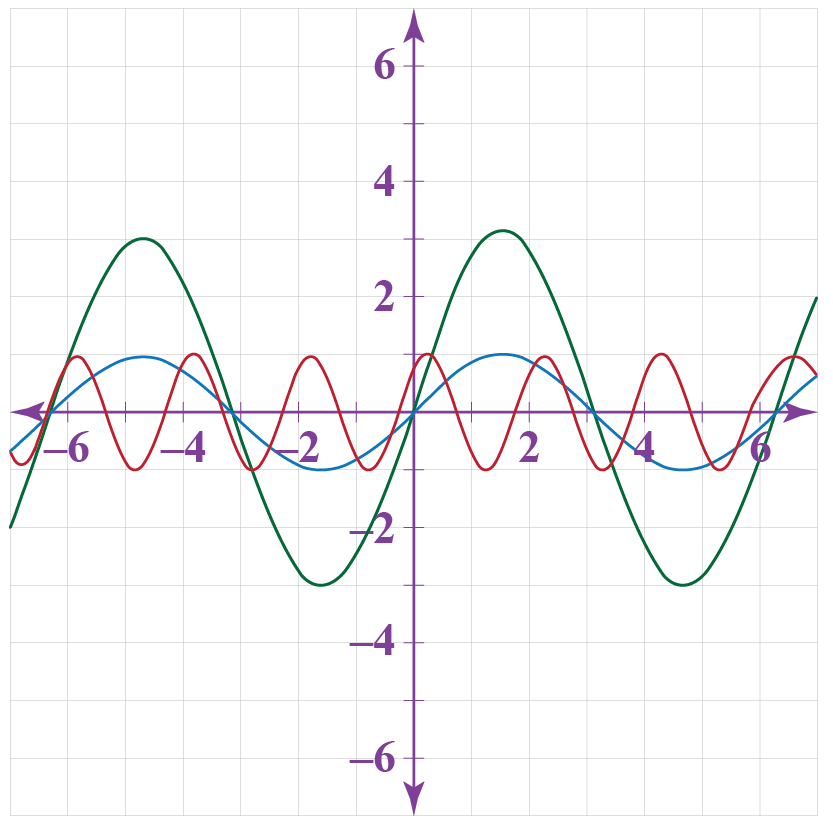In this section, we will learn about vertical scaling in detail and practice solving questions around it.

You can check out the interactive simulations to know more about the lesson and try your hand at solving a few interesting interactive questions at the end of the page.

## Lesson Plan

 1 What Do You Mean by Vertical Scaling? 2 Important Notes on Vertical Scaling 3 Solved Examples on Vertical Scaling 4 Challenging Questions on Vertical Scaling 5 Interactive Questions on Vertical Scaling

## What Do You Mean by Vertical Scaling?

Scaling is a process of changing the shape and size of the graph of the function.

Vertical scaling refers to changing the shape and size of the graph of the function along the y-axis and is done by multiplying the function by some constant.

Suppose, we have a function, $$y = f(x)$$

Vertical scaling of the above function can be done just by multiplying the function with a constant C, i.e.,

$y = Cf(x)$

Example

We have a function $$y = f(x)$$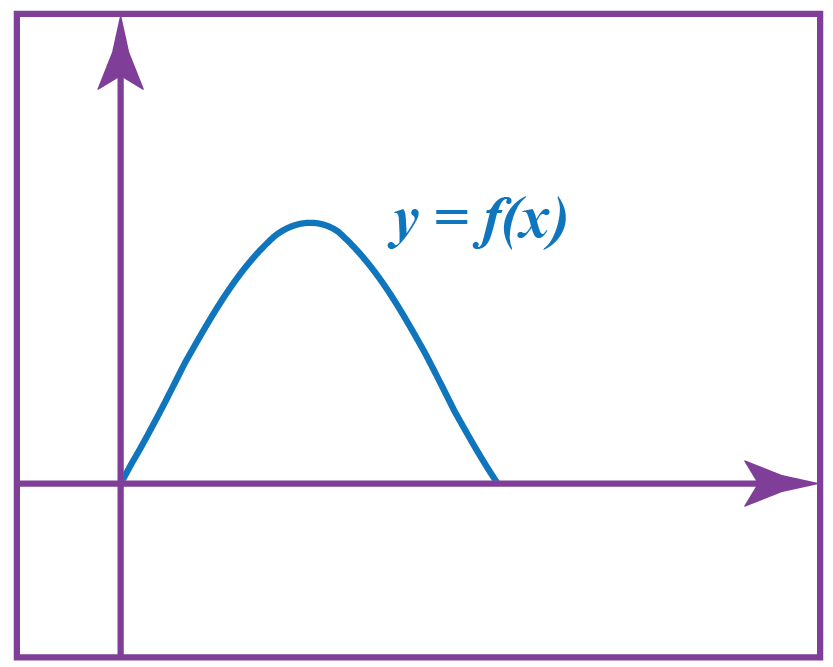We have multiplied the function with 2, i.e., $y = 2f(x)$

We get,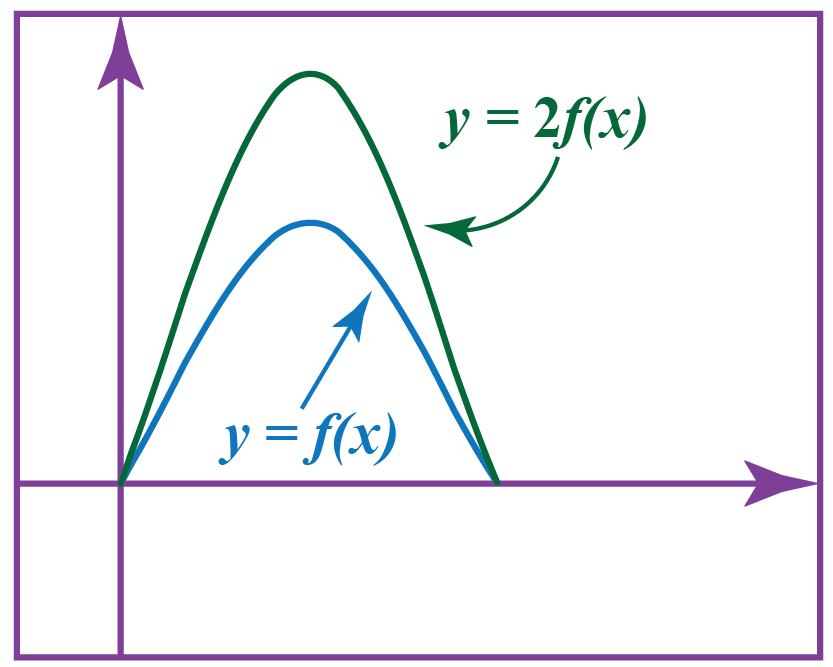The distance of the points on the curve gets farther away from the x-axis.

The shape of the curve depends on the value of C:

• If C > 1, the graph stretches and makes the graph steeper.
• If C < 1, the graph shrinks and makes the graph flatter.
The point on f(x) The point on y = Cf(x)
$$(x, y)$$

$$(x, Cy )$$

## How to Do Vertical Scaling?

Let us understand this by an example:

Example

Suppose we have a basic quadratic equation $$f(x) = x^2$$ and the graphical representation of the graph is shown below.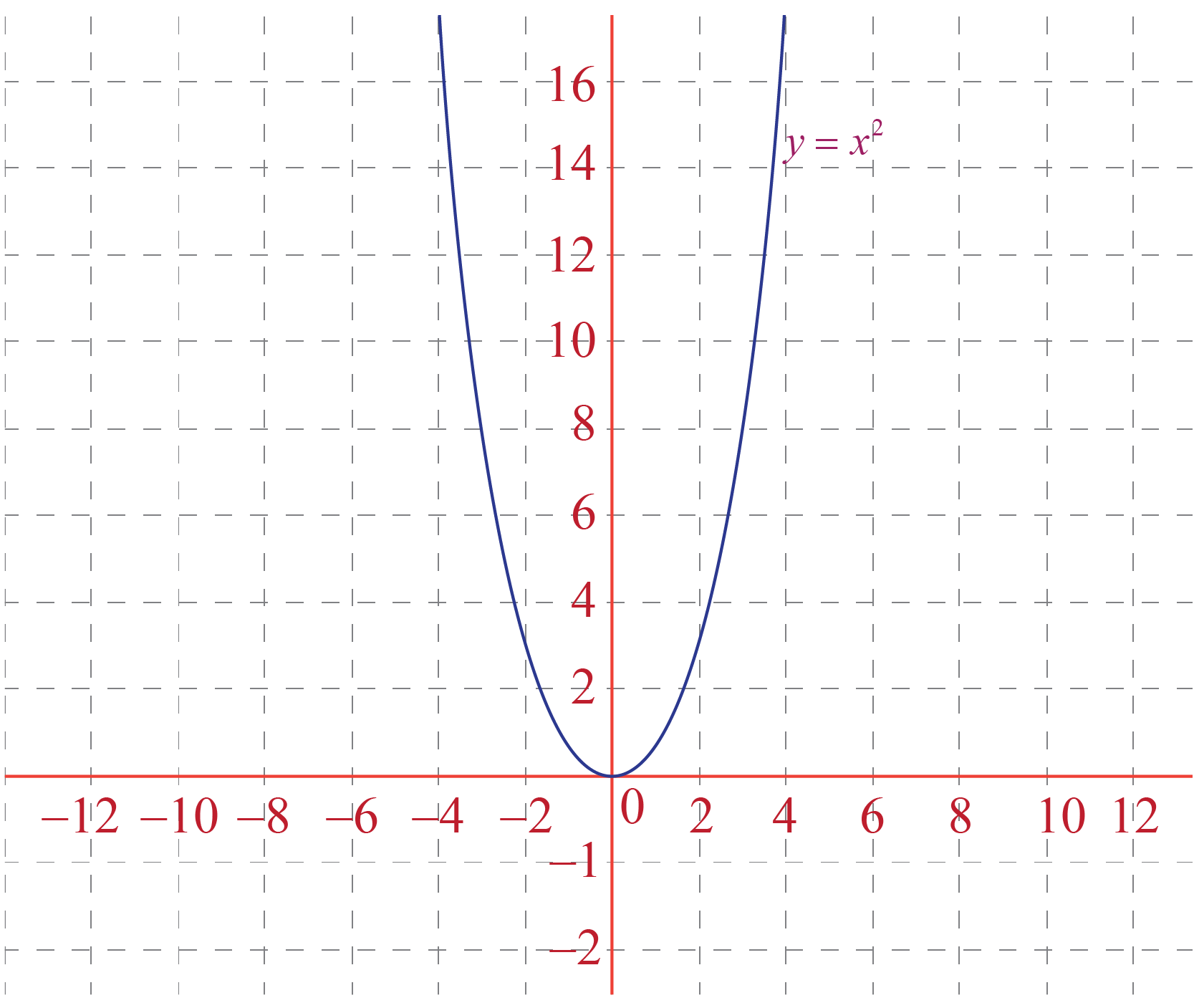Now if we want to vertically scale this graph, we have to follow the given steps:

Steps

Step 1: Select the constant by which we want to scale the function.

Here we have selected $$\color{orange}{+2}$$.

Step 2: Write the new function as $$g(x) = Cf(x)$$, where C is the constant.

Here, our equation of the new function will be

$g(x) = \color{orange}{2} f(x) = \color{orange}{2} x^2$

Step 3: Trace the new function graph by replacing each value of y by Cy.

Here we have to replace the value of y-coordinate by $$\color{orange}{2}y$$.

Y coordinate of each point in the graph is multiplied by $$\pm C$$, and the curve shrinks/stretches accordingly.

Here, we have the graph of $$x^2$$ and it is stretched by a factor of $$\color{orange}{+2}$$ units in the y-direction.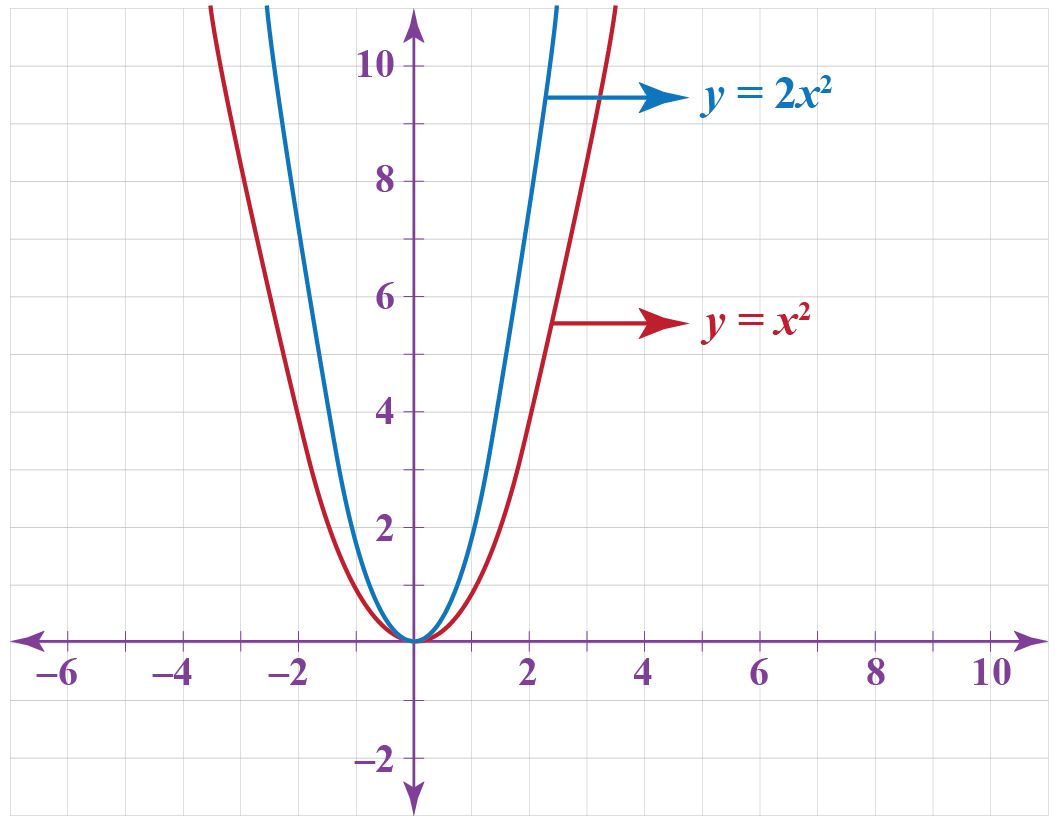Here, we have got $$\color{orange}{+2}$$ units vertically scaled graph of $$x^2$$ or of the function $$g(x) = 2x^2$$.

Note: as we have scaled it with a factor of $$\color{orange}{+2}$$ units, it has made the graph steeper.

## Vertical Scaling in Graphs

Vertical scaling of various graphs of the functions are shown below:

• Vertical scaling of function f(x) = x+2 by a factor of 2 units is shown in the graph below: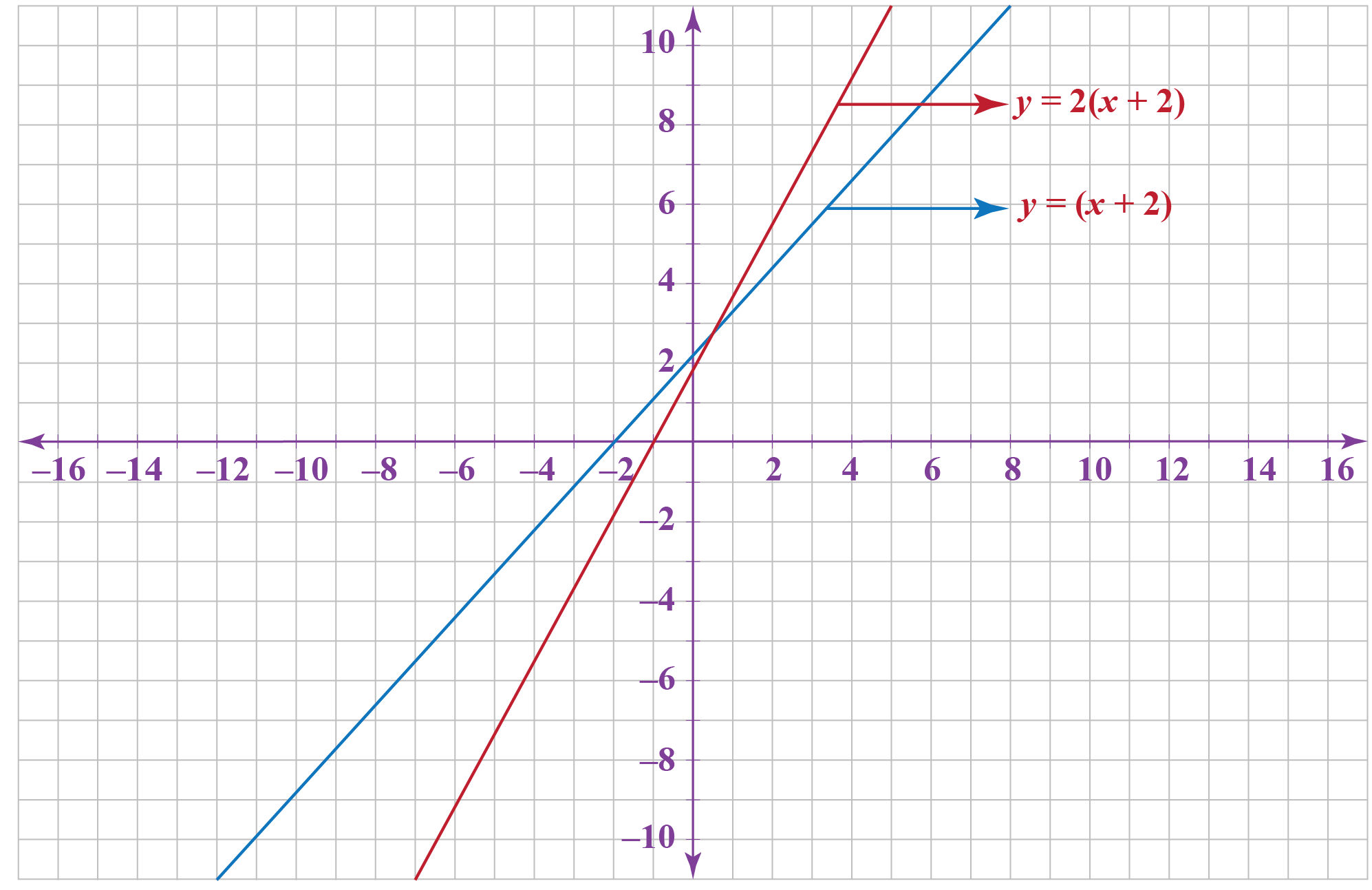• Vertical scaling of function $$f(x) =(x^2 +3x+2)$$ by a factor of -4 units is shown in the graph below: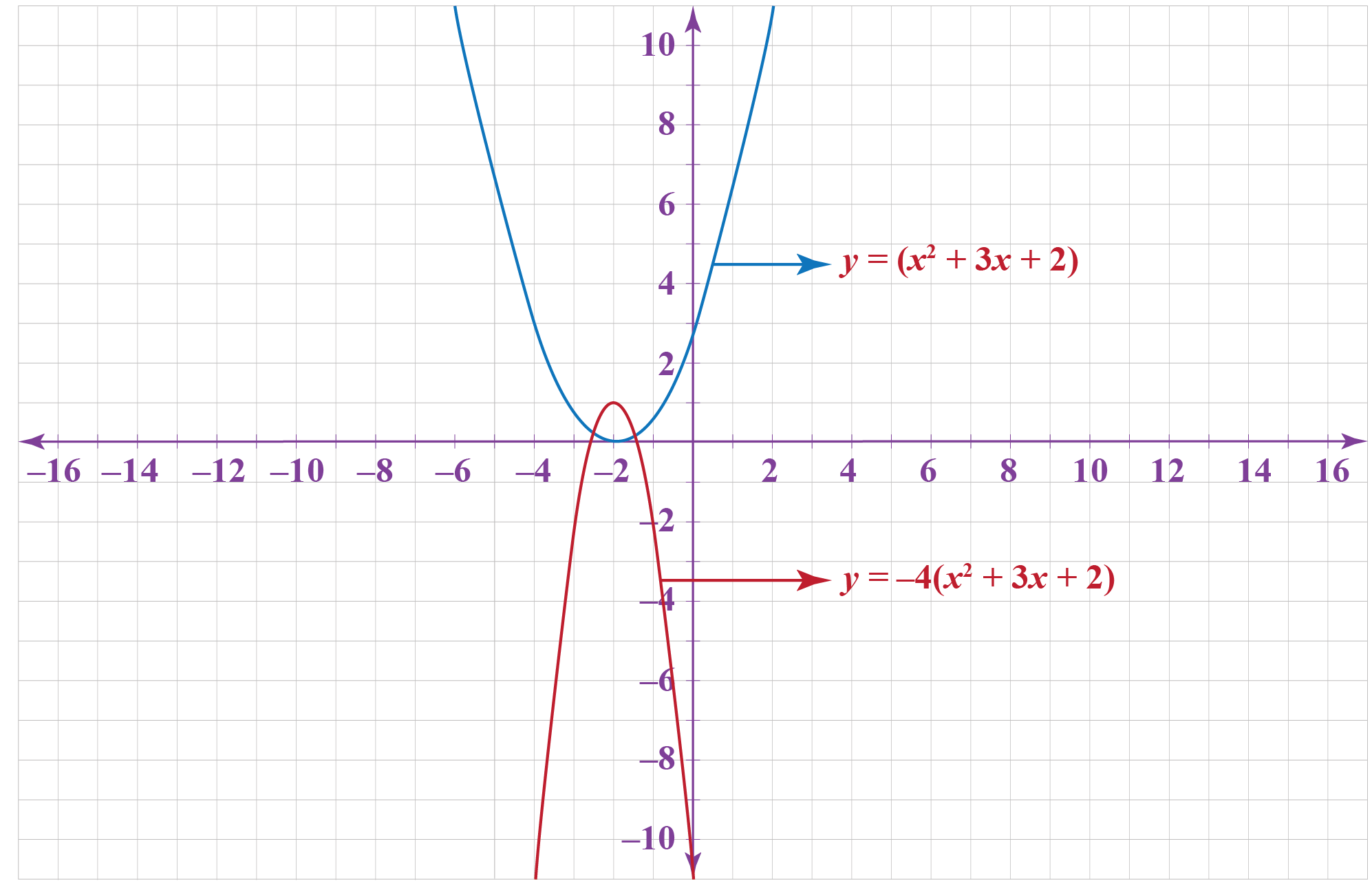• Vertical scaling of function $$f(x) = \sin x$$ by a factor of 3 units is shown in the graph below: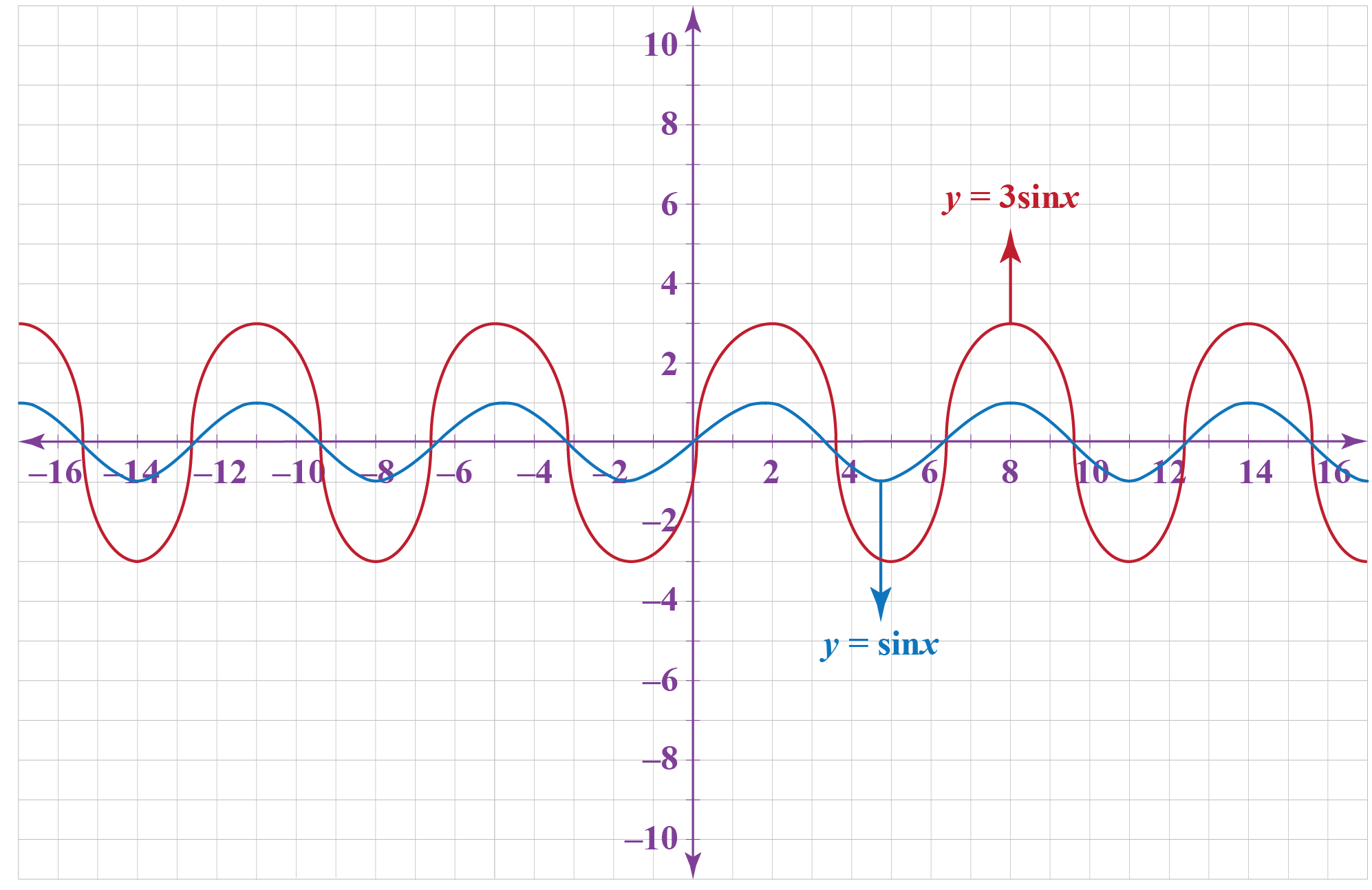1. Vertical scaling refers to the shrinking or stretching of the curve along the y-axis by some specific units.
2. Vertical scaling of function f(x) is given by g(x) =  $$\pm$$ C f(x).
Functions
Functions
grade 10 | Questions Set 2
Functions
grade 10 | Questions Set 1
Functions
More Important Topics
Numbers
Algebra
Geometry
Measurement
Money
Data
Trigonometry
Calculus
More Important Topics
Numbers
Algebra
Geometry
Measurement
Money
Data
Trigonometry
Calculus
Learn from the best math teachers and top your exams

• Live one on one classroom and doubt clearing
• Practice worksheets in and after class for conceptual clarity
• Personalized curriculum to keep up with school